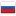Please subscribe to the official Codeforces channel in Telegram via the link https://t.me/codeforces_official. ×

Разбор Codeforces Round #382

Revision en3, by albertg, 2016-11-29 01:27:11

735A - Ostap and Grasshopper

Problem on programming technique. You have to find at which positions are grasshoper and insect. If k does not divide the difference of position, then answer is NO. Otherwise we have to check positions pos+k, pos+2k, ..., where pos is the minimal poisiton of grasshoper and insect. If somewhere is an obstacle, then answer is NO, otherwise the answer is YES.

735B - Urbanization

First of all, note that n1+n2 chosen ones should be people with top (n1+n2) coeficients. Secondly, if the person with intelegence C will be in the first city then he will contribute to our overall IQ with C/n1 points. So, if n1<n2, then top-n1 ratings should be in the small city and the top-n2 from others — in the big city.

736A - Tennis Championship

Let us solve the inverse problem: at least how many competitors should be, if the champion will have n matches. Then there's obvious reccurrent formula: f(n+1)=f(n)+f(n-1) (Let us make the draw in a way, where the champion will play n matches to advance to finals and the runner-up played (n-1) matches to advance the final). So, we have to find the index of maximal fibunacci number which is no more that number in the input.

736B - Taxes

The first obvious fact is that the answer for prime numbers is 1. If the number is not prime, then the answer is at least 2. When is it possible? It is possible in 2 cases; when it is sum of 2 primes of its maximal divisor is 2. If 2 divides n, then so does integer n/2. n/2<=2=>n<=4=>n=4, where n is prime. According to Goldbach's conjecture, which is checked for all numbers no more than 10^9, every number is a sum of two prime numbers. Odd number can be sum of two primes, if (n-2) is prime (the only even prime number is 2). Otherwise, the answer is 3 — n=3+(n-3), (n-3) is sum of 2 primes, because it is even.

736C - Ostap and Tree

First of all, thanks to albert96 and GlebsHP for their help with the tutorial of this problem. Secondly, sorry for being late.

Problem can be solved by the method of dynamic programming. Let dp[v][i][j] be the number of possibilities to color subtree of vertex v in such a way that the closest black vertex is on depth i, and the closest white vertex — on depth j (we also store dp[v][-1][j] and dp[v][i][-1] in the cases where there are no black and white vertexes in diapason k of v respectively). In order to connect two subtrees, we can check all pairs (i,j) in both subtrees (by brute-force algorithm). Then let we have pair (a,c) in the first subtree and pair (b,d) in the second one. If min(a,c)+max(b,d)<=k, then we update value of current vertex.

Complexity of the algorithm O(n*k^4), which is acceptable for this particular problem (n — the number of vertexes, k^4 brute force search of pairs (a,b); (c,d)).

736D - Permutations

This problem consists of 3 ideas. Idea 1: remainder modulo 2 of the number of permutation is equal to the remainder modulo 2 of the determinant of the matrix whose entries are 1 if (ai,bi) is in our list and 0 otherwise. Idea 2: If we cahnge 1 by 0, then the determinant will differ by algebraic compliment. That is, if we count inverse matrix, than it will reflect reminders modulo 2 (B(m,n)=A'(m,n)/detA, detA is odd). Idea 3: Inverse matrix can be counted for O((n/32)^3) time. However, we can work is field of integers modulo 2. The summation can be replaced by XOR. So if we store in one "int" not a single but 32 numbers, then we can reduce our assymptocy to O(n^3/32), which is OK.

736E - Chess Championship

Suppose set (a1,a2,...,am). Then the list is valid if set {2m-2, 2m-4, 2m-6, ..., 0} majorizes the set {a1,a2,...,am}. Let us prove it! Part 1: Suppose n<=m. Top n players will play n(n-1)/2 games with each other and n(m-n) games with low-ranked contestants. In these games they will collect 2*n(n-1)/2 points (in each game there is exactly 2 points) for sure and at most 2*n*(m-n) points in games with others. So they will have at most 2*(n*(n-1)/2+n*(m-n))=2*((m-1)+(m-2)+...+(m-n)) points. Now construction: Let's construct results of participant with most points and then use recursion. Suppose the winner has even number of points (2*(m-n) for some n). Then we consider that he lost against contestants holding 2,3,4,...,n places and won against others. If champion had odd number of points (2*(m-n)-1 for some n), then we will construct the same results supposing that he draw with (n+1)th player instead of winning agianst him. It is easy to check that majorization is invariant, so in the end we will have to deal with 1 men competition, when set of scores {a1} is majorized by set {0}. So a1=0, and there is obvious construction for this case. So we have such an algorithm: we search for a compiment set which is majorized by {2m-2,2m-4,...,0}. If there is no such set answer is NO. Otherwise answer is YES and we construct our table as shown above. Assymptosy is O(m^2logm) (calling recursion m times, sorting the array (we can lose non-decreasing order because of poor results) and then passing on it linearly.

#### History

RevisionsRev. Lang. By When Δ Comment
en3albertg 2016-11-29 01:27:11 46
ru4albertg 2016-11-29 01:25:20 18
en2albertg 2016-11-28 22:40:13 924
ru3albertg 2016-11-28 22:32:28 862
ru2albertg 2016-11-27 23:42:58 6 Мелкая правка: 'тотика: O(n^2logn) (n раз мы вы' -> 'тотика: O(m^2logm) (m раз мы вы'
en1albertg 2016-11-27 23:40:58 4299 Initial revision for English translation
ru1albertg 2016-11-27 22:56:18 4907 Первая редакция (опубликовано)